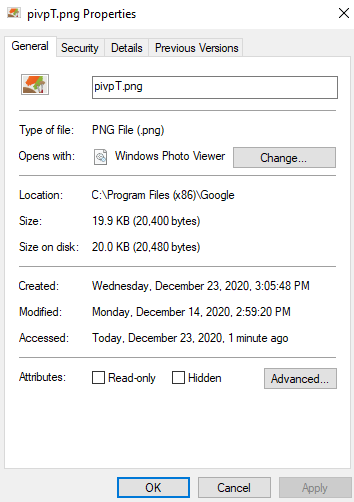Related Articles

# How to get file creation and modification date or time in Python?

• Last Updated : 03 Jan, 2021

A Timestamp is a sequence of characters, that signify the occurrence of an event. Timestamps are required extensively in computer science. This exists of varying precision and accuracy i.e. some timestamps have the precision up to milliseconds for the occurrence of the even, others do not. This allows Timestamps of different forms (and standards) to exist. In this article, we will take a look at methods for finding creation and modification timestamps of a file. We will be using the file with the following properties for the demonstration.We will use getctime() and getmtime() function found inside path module in the os library, for getting the creation and modification times of the file. Both the above function return time in seconds since EPOCH (00:00:00 UTC on 1 January 1970) (time is of float datatype). Since that number doesn’t resemble an understandable timestamp, we would have to convert that time i.e. it becomes recognizable. For that purpose, we would be using ctime() function found inside the time library.

ctime(seconds)

Convert a time in seconds since the Epoch to a string in local time.

This is equivalent to asctime(localtime(seconds)). When the time tuple is

not present, current time as returned by localtime() is used.

Parameter:

seconds = A integer/float value

Return:

A string denoting a timestamp

Below is the implementation:

## Python3

 `import` `os``import` `time`` ` `# Path to the file/directory``path ``=` `r``"C:\Program Files (x86)\Google\pivpT.png"`` ` `# Both the variables would contain time ``# elapsed since EPOCH in float``ti_c ``=` `os.path.getctime(path)``ti_m ``=` `os.path.getmtime(path)`` ` `# Converting the time in seconds to a timestamp``c_ti ``=` `time.ctime(ti_c)``m_ti ``=` `time.ctime(ti_m)`` ` `print``(``    ``f"The ``file` `located at the path {path}``    ``was created at {c_ti} ``and` `was last modified at {m_ti}")`

Output:

The file located at the path C:\Program Files (x86)\Google\pivpT.png was created at Wed Dec 23 15:05:48 2020

The timestamp of the above code has the following format qualifiers –

`[Day](3) [Month](3) [day](2) [Hours:Minutes:Seconds](8) [Year](4)`

Where the word inside the bracket is the cue for what is being displayed, and the number following it within the parenthesis displays the length it will occupy.

Note: It is possible to alter the format of the timestamp used in the above code. By default, the ctime() function would return a timestamp of the aforementioned syntax. In order to change it, we would have to pass it to strptime() function (also found inside time library) to create a time structure (object) out of it. Then we can pass format specifiers to strftime(), to create a custom timestamp out of the time structure. In the following code, we will be getting the modification time of the same file in ISO 8601 timestamp format.

## Python3

 `import` `os``import` `time`` ` `path ``=` `r``"C:\Program Files (x86)\Google\pivpT.png"`` ` `ti_m ``=` `os.path.getmtime(path)`` ` `m_ti ``=` `time.ctime(ti_m)`` ` `# Using the timestamp string to create a ``# time object/structure``t_obj ``=` `time.strptime(m_ti)`` ` `# Transforming the time object to a timestamp ``# of ISO 8601 format``T_stamp ``=` `time.strftime(``"%Y-%m-%d %H:%M:%S"``, t_obj)`` ` `print``(f``"The file located at the path {path} was last modified at {T_stamp}"``)`

Output: